# Water is flowing into the top of a tank at a rate of 200 gpm. The...

Water is flowing into the top of a tank at a rate of 200 gpm. The tank is 18 inches in diameter and has a 3 inch diameter hole in the bottom, through which the water flows out. If the inflow rate is adjusted to match the outflow rate, what will the height of the water be in the tank if friction is negligible?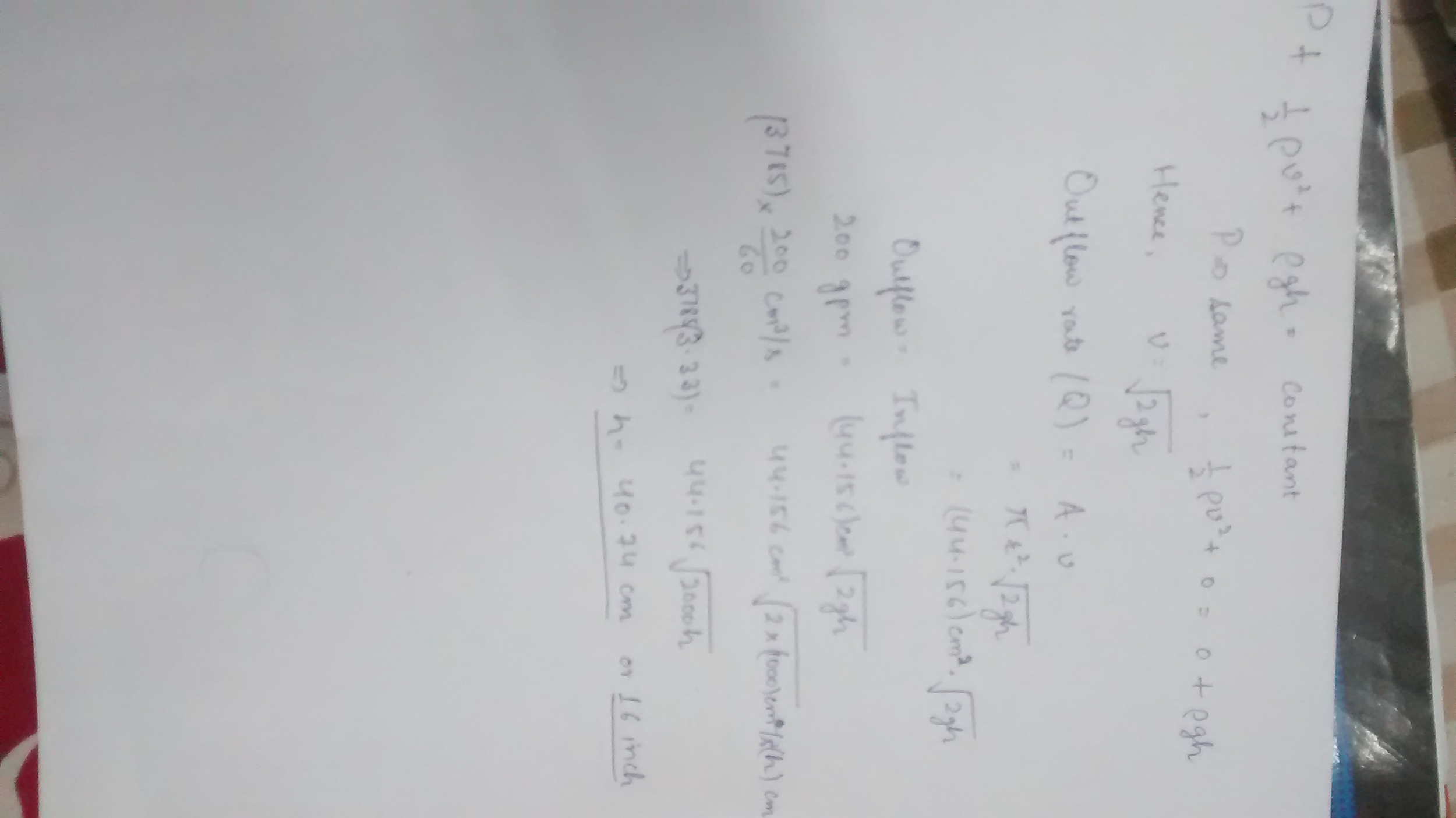#### Earn Coin

Coins can be redeemed for fabulous gifts.

Similar Homework Help Questions
• ### 7 Water is flowing into the top of an open cylindrical tank (diameter D) at a...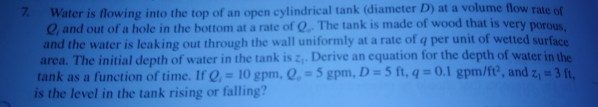7 Water is flowing into the top of an open cylindrical tank (diameter D) at a volume flow rate of e and out of a hole in the bottom at a rate of O The tank is made of wood that is very porous and the water is leaking out through the wall uniformly at a rate of q per unit of wetted surface area. The initial depth of water in the tank is 2,. Derive an equation for the...

• ### 3. (3 points) A tank of diameter D is filled with water up to a height...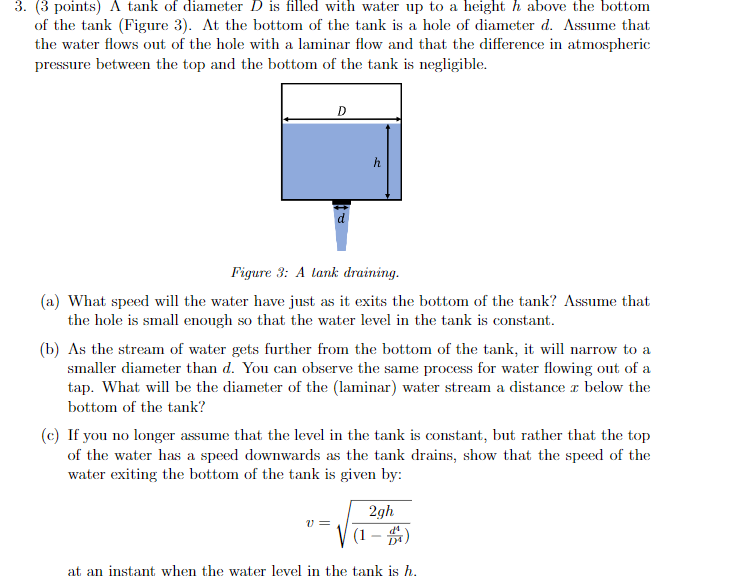3. (3 points) A tank of diameter D is filled with water up to a height h above the bottom of the tank (Figure 3). At the bottom of the tank is a hole of diameter d. Assume that the water flows out of the hole with a laminar flow and that the difference in atmospheric pressure between the top and the bottom of the tank is negligible Figure 3: A lank draining a) What speed will the water have...

• ### L 2. Steady statemass balance: Water is flowing at steady state in a 0.1 meter-diameter pipe...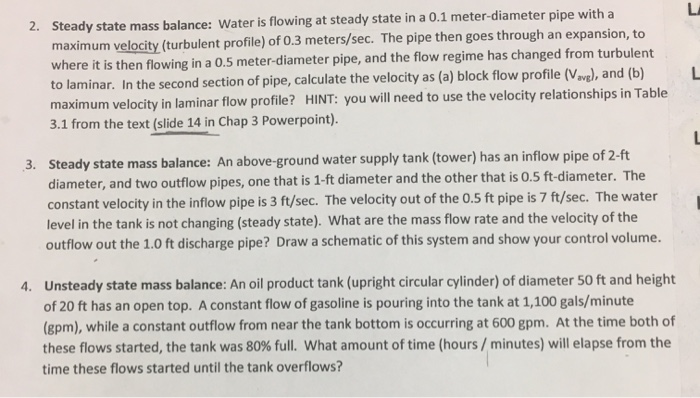L 2. Steady statemass balance: Water is flowing at steady state in a 0.1 meter-diameter pipe with a maximum velocity (turbulent profile) of 0.3 meters/sec. The pipe then goes through an expansion, to where it is then flowing in a 0.5 meter-diameter pipe, and the flow regime has changed from turbulent to laminar. In the second section of pipe, calculate the velocity as (a) block flow profile (Vavg), and (b) maximum velocity in laminar flow profile? HINT: you will need...

• ### (3 points) A tank of diameter D is filled with water up to a height h...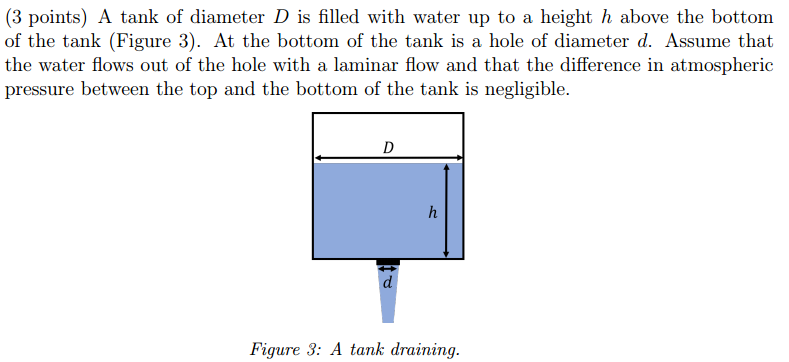(3 points) A tank of diameter D is filled with water up to a height h above the bottom of the tank (Figure 3). At the bottom of the tank is a hole of diameter d. Assume that the water flows out of the hole with a laminar flow and that the difference in atmospheric pressure between the top and the bottom of the tank is negligible. Figure 3: A tank draining (c) If you no longer assume that the...

• ### Water flows at a rate of 30 gpm through a vertical pipe that changes size and...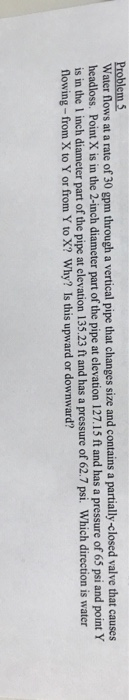Water flows at a rate of 30 gpm through a vertical pipe that changes size and contains a partially-closed valve that causes headloss. Point X is in the 2-inch diameter part of the pipe at elevation 127.15 ft and has a pressure of 65 psi and point Y is in the I inch diameter part of the pipe at elevation 135.23 ft and has a pressure of 62.7 psi. Which direction is water flowing-from X to Y or from Y...

• ### Draining of cylindrical tank. You have a cylindrical tank full of water with a diameter =Dtank....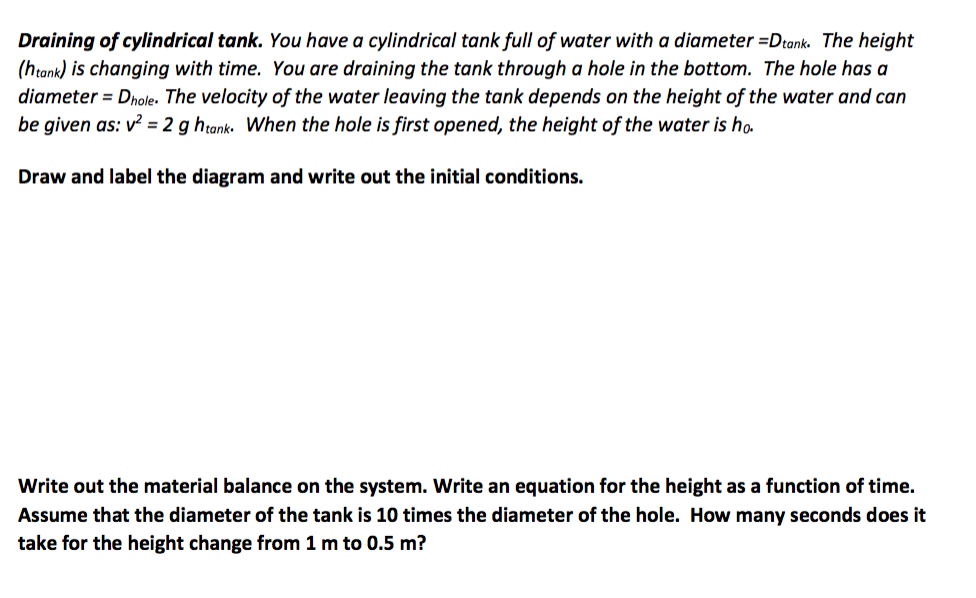Draining of cylindrical tank. You have a cylindrical tank full of water with a diameter =Dtank. The height (htank) is changing with time. You are draining the tank through a hole in the bottom. The hole has a diameter Dhole. The velocity of the water leaving the tank depends on the height of the water and can be given as: v2 = 2 g htank. When the hole is first opened, the height of the water is ho. Draw and...

• ### 1. Atall cylindrical tank, held above ground on stilts, is partially filled with water. (See top...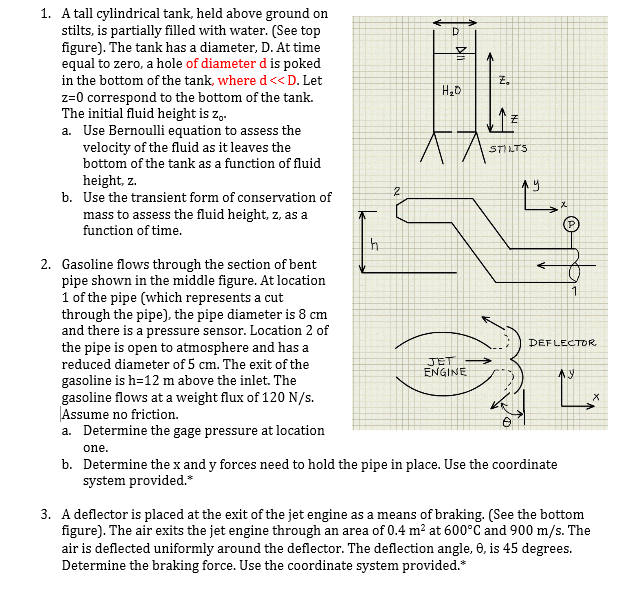1. Atall cylindrical tank, held above ground on stilts, is partially filled with water. (See top figure). The tank has a diameter, D. At time equal to zero, a hole of diameter d is poked in the bottom of the tank, where d<< D. Let z-0 correspond to the bottom of the tank. The initial fluid height is za. Had Use Bernoulli equation to assess the velocity of the fluid as it leaves the bottom of the tank as a...

• ### A jet of water squirts out horizontally from a hole near the bottom of the tank...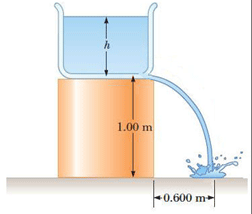A jet of water squirts out horizontally from a hole near the bottom of the tank shown in the figure. If the hole has a diameter of 4.00 mm, what is the height h of the water level in the tank? A tank of water sits upright on a surface that is elevated above the ground. The height of the elevated surface above the ground is labeled 1.00 m. The height of the water from the bottom of the tank...

• ### DIfferential equation conical draining tank

A right circular conical tank loses water out of a hole at its bottom.Part A:Determine a differential Equation for the height of the water h at time t. The radius of the hole is 2 inches, g= 32 ft/s^2 and the friction factor is .6Dv/dt = -cA(sub h)(2gh)^1/2h of tank = 20 ftradius of top of tank = 8 ftPart b Find the time required to empty the tankWill rate A+ thanks in advance

• ### the water level. If the rate of flow from the leak is equal 16.0 m below 2.50 X 10-3 m3/min, determine (a) the speed...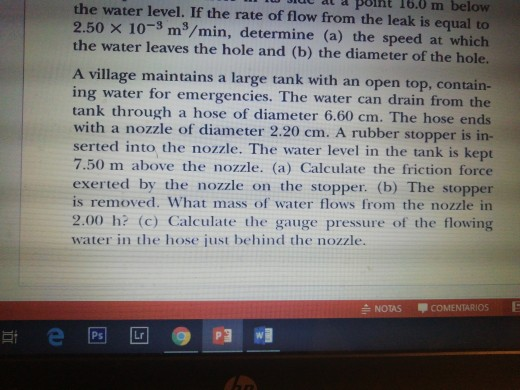the water level. If the rate of flow from the leak is equal 16.0 m below 2.50 X 10-3 m3/min, determine (a) the speed the water leaves the hole and (b) the diameter of the hole. to at which A village maintains a large tank with an open top, contain- ing water for emergencies. The water can drain from the tank through a hose of diame ter 6.60 cm. The hose ends with a nozzle of diameter 2.20 cm. A...